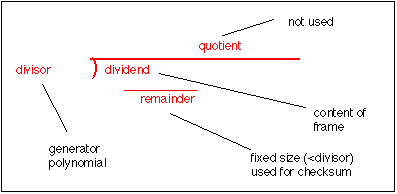# Cyclic Redundancy Checks (CRC)

A Cyclic Redundancy Check (CRC) is a form of Integrity Check.

## Cyclic Redundancy Checks

A powerful method for detecting errors in the received data is by grouping the bytes of data into a block and calculating a CRC. This is usually done by the link protocol and the calculated CRC is appended to the end of a link frame.

The CRC is calculated by performing a modulo 2 division of the data by a generator polynomial and recording the remainder after division.Three polynomials are in common use they are:

• CRC-16 = x16 + x15 + x2+ 1 (used in HDLC)
• CRC-CCITT = x16 + x12 + x5 + 1
• CRC-32 = x32 + x26 + x23 + x22 + x16 + x12 + x11 + x10 + x8 + x7 + x5 + x4 + x2 + x + 1 (used in Ethernet)

Although this division may be performed in software, it usually performed using a shift register and X-OR gates. The hardware solution for implementing a CRC is much simpler than a software approach. One example for a CRC-16 is:Basic Encoder/Decoder for a 16-bit CRC

## CRC Decoder

A practical implementation of a decoder also requires a method to initialise the encoder prior to transmission of the first bit of data in a frame, and to flush the encoder after sending the last byte. In the example below (which uses a different representation of the schematics for X-OR gates and shift register elements), the process starts by initialising the encoder with zero bits, by setting the switch to B. Some CRC's initialise the register to a non-zero value, which can give added detection capability when the first set of bits in a frame may themselves be zero. Then the switch is moved to position A and one data bit enter the encoder for each clock cycle. The data bits are immediately available at the output. After the last bit has been sent, the switch is returned to position B and the contents of the encoder are sent to the output. This is often called flushing the encoder and requires one clock cycle per bit held in the shift register.Diagram of suggested implementation of an Encoder/Decoder

On reception, the process is reversed. The CRC register is first set to zero (or the initial value on transmission, if non-zero). The bits (this time including the CRC) are fed into the register on each clock cycle. If the CRC contains the value zero (assuming initialisation was zero), the CRC is valid, if not it has detected an error. The CRC-16 is able to detect all single errors, all double errors, all odd numbers of errors and all errors with burst less than 16 bits in length. In addition 99.9984 % of other error patterns will be detected.

## Bit Order

The CRC is the only field which is by convention sent most significant bit first. (This is contrary to all header and payload bytes which are sent least significant bit first.) Thus the first bit of the a CRC-16 to be sent is the bit corresponding to X16 and the last, the bit corresponding to X1.